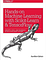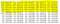# Predict Stock Prices Using Python & Machine Learning

Using Python, Linear Regression & Support Vector Regression

In this article I will show you how to write a python program that predicts the price of stocks using two different machine learning algorithms, one is called a Support Vector Regression (SVR) and the other is Linear Regression. So you can start trading and making money ! Actually this program is really simple and I doubt any major profit will be made from this program, but it may be slightly better than guessing!

It is extremely hard to try and predict the direction of the stock market, but in this article I will give it a try. Even people with a good understanding of statistics and probabilities have a hard time doing this.

A Support Vector Regression (SVR) is a type of Support Vector Machine, and is a type of supervised learning algorithm that analyzes data for regression analysis. In 1996, this version of SVM for regression was proposed by Christopher J. C. Burges, Vladimir N. Vapnik, Harris Drucker, Alexander J. Smola and Linda Kaufman. The model produced by SVR depends only on a subset of the training data, because the cost function for building the model ignores any training data close to the model prediction.

# Support Vector Machine Pros:

1. It is effective in high dimensional spaces.
2. It works well with clear margin of separation.
3. It is effective in cases where number of dimensions is greater than the number of samples.

# Support Vector Machine Regression Cons:

1. It does not perform well, when we have large data set.
2. Low performance if the data set is noisy ( a large amount of additional meaningless information).

# Types Of Kernel:

1. linear
2. polynomial
4. sigmoid

Linear regression is a linear approach to modeling the relationship between a scalar response (or dependent variable) and one or more explanatory variables (or independent variables).

# Linear Regression Pros:

1. Simple to implement.
2. Used to predict numeric values.

# Linear Regression Cons:

1. Prone to overfitting.
2. Cannot be used when the relation between independent and dependent variable are non linear.

If you prefer not to read this article and would like a video representation of it, you can check out the YouTube Video below. It goes through everything in this article with a little more detail, and will help make it easy for you to start programming your own Machine Learning model even if you don’t have the programming language Python installed on your computer. Or you can use both as supplementary materials for learning about Machine Learning !

If you are also interested in reading more on machine learning to immediately get started with problems and examples then I strongly recommend you check out Hands-On Machine Learning with Scikit-Learn and TensorFlow: Concepts, Tools, and Techniques to Build Intelligent Systems. It is a great book for helping beginners learn how to write machine learning programs, and understanding machine learning concepts.Hands-On Machine Learning with Scikit-Learn and TensorFlow: Concepts, Tools, and Techniques to Build Intelligent Systems

# Start Programming:

I will start by stating what I want this program to do. I want this program to predict the prices of a stock 30 days in the future based off of the current Adjusted Close price.

First I will import the dependencies, that will make this program a little easier to write. I’m importing the machine learning library sklearn, quandl, and numpy.

`#Install the dependenciesimport quandlimport numpy as np from sklearn.linear_model import LinearRegressionfrom sklearn.svm import SVRfrom sklearn.model_selection import train_test_split`

Next I will get the stock data from quandl, and take a look at the data set. Here I am getting Amazon stock data aka AMZN, storing it into a variable called ‘df’ which is short for data frame, and printing the first 5 rows of data.

`# Get the stock datadf = quandl.get("WIKI/AMZN")# Take a look at the dataprint(df.head())`

I only need the Adjusted Close (Adj. Close) price, so I am getting data only from the column ‘Adj. Close’ and storing this back into the variable ‘df’. Then printing the first 5 rows of the new data set.

`# Get the Adjusted Close Price df = df[['Adj. Close']] # Take a look at the new data print(df.head())`

Now , I’m creating a variable called forecast_out, to store the number of days (30 days) into the future that I want to predict. This variable will be used through out the program so that I can simply change the number and the rest of the program will correspond accordingly. So if I decide I only want to look 20 days into the future, I can simply change this variable from 30 to 20, and the program will predict now 20 days into the future.

I also need a column (the target or dependent variable) that will hold the predicted price values 30 days into the future. The future price that I want that’s 30 days into the future is just 30 rows down from the current Adj. Close price. So I will create a new column called ‘Prediction’ and populate it with data from the Adj. Close column but shifted 30 rows up to get the price of the next 30 days, and then print the last 5 rows of the new data set.

Note: Since I shifted the data up 30 rows, the last 30 rows of data for the new column ‘Prediction’ will be empty or contain the value ‘NaN’ (Not A Number).

`# A variable for predicting 'n' days out into the futureforecast_out = 30 #'n=30' days#Create another column (the target ) shifted 'n' units updf['Prediction'] = df[['Adj. Close']].shift(-forecast_out)#print the new data setprint(df.tail())`

Next, I want to create the independent data set (X). This is the data set that I will use to train the machine learning model(s). To do this I will create a variable called ‘X’ , and convert the data into a numpy (np) array after dropping the ‘Prediction’ column, then store this new data into ‘X’.

Then I will remove the last 30 rows of data from ‘X’, and store the new data back into ‘X’. Last but not least I print the data.

`### Create the independent data set (X)  ######## Convert the dataframe to a numpy arrayX = np.array(df.drop(['Prediction'],1))#Remove the last '30' rowsX = X[:-forecast_out]print(X)`

I created the independent data set in the previous step, now I will create the dependent data set called ‘y’. This is the target data, the one that holds the future price predictions.

To create this new data set ‘y’, I will convert the data frame into a numpy array and from the ‘Prediction’ column, store it into a new variable called ‘y’ and then remove the last 30 rows of data from ‘y’. Then I will print ‘y’ to make sure their are no NaN’s.

`### Create the dependent data set (y)  ###### Convert the dataframe to a numpy array y = np.array(df['Prediction'])# Get all of the y values except the last '30' rowsy = y[:-forecast_out]print(y)`

Now that I have my new cleaned and processed data sets ‘X’ & ‘y’. I can split them up into 80% training and 20 % testing data for the model(s).

`# Split the data into 80% training and 20% testingx_train, x_test, y_train, y_test = train_test_split(X, y, test_size=0.2)`

I can start creating and training the models ! First I will create and train the Support Vector Machine (Regression).

`# Create and train the Support Vector Machine (Regressor) svr_rbf = SVR(kernel='rbf', C=1e3, gamma=0.1) svr_rbf.fit(x_train, y_train)`

Let’s test the model by getting the score also known as the coefficient of determination R² of the prediction. The best possible score is 1.0, and the model returns a score of 0.9274190417518909.

`# Testing Model: Score returns the coefficient of determination R^2 of the prediction. # The best possible score is 1.0svm_confidence = svr_rbf.score(x_test, y_test)print("svm confidence: ", svm_confidence)`

Next I will create & train the Linear Regression model !

`# Create and train the Linear Regression  Modellr = LinearRegression()# Train the modellr.fit(x_train, y_train)`

Let’s test the model by getting the score also known as the coefficient of determination R² of the prediction. The best possible score is 1.0, and the model returns a score of 0.9874918531515935.

`# Testing Model: Score returns the coefficient of determination R^2 of the prediction. # The best possible score is 1.0lr_confidence = lr.score(x_test, y_test)print("lr confidence: ", lr_confidence)`

Looks like in this case the Linear Regression model will be better to use to predict the future price of Amazon stock, because it’s score is closer to 1.0.

Now I am ready to do some forecasting / predictions. I will take the last 30 rows of data from the data frame of the Adj. Close price, and store it into a variable called x_forecast after transforming it into a numpy array and dropping the ‘Prediction’ column of course. Then I will print the data to make sure the 30 rows are all there.

`# Set x_forecast equal to the last 30 rows of the original data set from Adj. Close columnx_forecast = np.array(df.drop(['Prediction'],1))[-forecast_out:]print(x_forecast)`

Finally, I have arrived at the moment of truth. I will print out the future price (next 30 days) predictions of Amazon stock using the linear regression model, and then print out the Amazon stock price predictions for the next 30 days of the support vector machine using the x_forecast data !

`# Print linear regression model predictions for the next '30' dayslr_prediction = lr.predict(x_forecast)print(lr_prediction)# Print support vector regressor model predictions for the next '30' dayssvm_prediction = svr_rbf.predict(x_forecast)print(svm_prediction)`Stock Predictions For The Next 30 Days. Highlighted in Yellow Is Linear Regression Predictions, Not Highlighted Is SVM Prediction